Search

Exponential Functions:
The "Natural" Exponential "
e" (page 5 of 5)

Sections: Introduction, Evaluation, Graphing, Compound interest, The natural exponentialThere is one very important number that arises in the development of exponential functions, and that is the "natural" exponential. (If you really want to know about this number, you can read the book "e: The Story of a Number", by Eli Maor.)

In the previous page's discussion of compound interest, recall that "n" stood for the number of compoundings in a year. What happens when you start compounding more and more frequently? What happens when you go from yearly to monthly to weekly to daily to hourly to minute-ly to second-ly to...?

Ignoring the principal, the interest rate, and the number of years by setting all these variables equal to "1", and looking only at the influence of the number of compoundings, we get:

 how often compounded computation yearly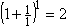semi-annually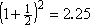quarterly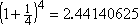monthly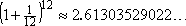weekly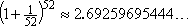daily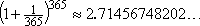hourly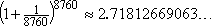every minute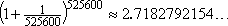every second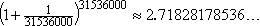As you can see, the computed value keeps getting larger and larger, the more often you compound. But the growth is slowing down; as the number of compoundings increases, the computed value appears to be approaching some fixed value. You might think that the value of the compound-interest formula is getting closer and closer to a number that starts out "2.71828". And you'd be right; the number we're approaching is called "e".

Advertisement

If you think back to geometry, you'll remember the number "pi", which was approximated by the decimal "3.14159" or the fraction " 22/7 ". Remember that we call pi by the name "pi" and use a symbol for this number because pi never ends when written as a decimal. It's not a "neat" number like 2 or 1/3; it is in fact an irrational number. But it's an important number; you'd have real trouble doing geometry without it. So we give this useful number the name "pi", to simplify our calculations and communication, because it's a lot easier to say "pi" than to say "3.141592653589 and so on forever" every time we need to refer to this number. We gave the number a letter-name because that was easier.

In the same way, this compound-interest number is also very useful. You may not see the usefulness of it yet, but it is vital in physics and other sciences, and you can't do calculus without it. As with pi, listing out its first dozen or so digits every time we refer to this number gets to be annoying, so we call it by the name "e".

The number "e" is the "natural" exponential, because it arises naturally in math and the physical sciences (that is, in "real life" situations), just as pi arises naturally in geometry. This number was discovered by a guy named Euler (pronounced "OY-ler"; I think he was Swiss), who described the number and named the number "e", and then swore that this stood for "exponential", and not for his own name.

Your calculator can do computations with e; it is probably a "second function" on your calculator, right above the "ln" or "LN" key on your calculator.

• Given f(x) = ex, evaluate f(3), rounding to two decimal places.
• I need to plug this into my calculator. (Check your owner's manual, if you're not sure of the key sequence.) I get:   Copyright © Elizabeth Stapel 2002-2011 All Rights Reserved

f(3) = 20.0855369232...

Rounded to two decimal places, the answer is f(3) = 20.09.

• Graph y = e2x.

 Since e is greater than 1, and since "2x" is "positive", then this should look like exponential growth. I will compute some plot points: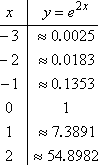Then I'll draw the graph: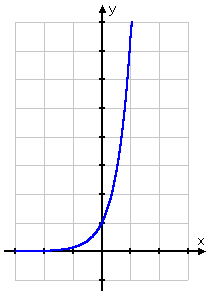Make sure, when you are evaluating e2x, that you format the expression correctly. Either multiply out the "2x" first, and then apply it to the e, or else put the "2x" inside parentheses. Otherwise, the calculator will think you mean "e2 × x", and will return the wrong values, as is demonstrated at right: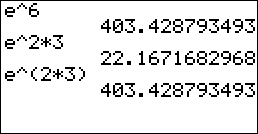Your teacher or book may go on at length about using other bases for growth and decay equations, but, in "real life" (such as physics), the natural base e is generally used. The equation for "continual" growth (or decay) is A = Pert, where "A", is the ending amount, "P" is the beginning amount (principal, in the case of money), "r" is the growth or decay rate (expressed as a decimal), and "t" is the time (in whatever unit was used on the growth/decay rate). Make sure you have memorized this equation, along with the meanings of all the variables. You are almost certain to see it again, especially if you are taking any classes in the sciences.

(This equation helped me pass a chemistry class. I really didn't know what the teacher was talking about, but all the test problems worked off this equation, so I just plugged in all the given information, and solved for whichever variable was left.  I'm not saying this to advocate being clueless in chemistry, but to demonstrate that the above really is a useful equation.)

The continuous-growth formula is first given in the above form "A = Pert", using "r" for the growth rate, but will later probably be given as A = Pekt, where "k" replaces "r", and stands for "growth (or decay) constant". Or different variables may be used, such as Q = Nekt, where "N" stands for the beginning amount and "Q" stands for the ending amount. The point is that, regardless of the letters used, the formula remains the same. And you should be familiar enough with the formula to recognize it, no matter what letters happen to be included within it.

• Certain bacteria, given favorable growth conditions, grow continuously at a rate of 4.6% a day. Find the bacterial population after thirty-six hours, if the initial population was 250 bacteria.
• As soon as I read "continuously", I should be thinking "continuously-compounded growth formula". "Continuously" is the buzz-word that tells me to use "A = Pert". The beginning amount was P = 250, the growth rate is r = 0.046, and the time t is 36/24 = 1.5 days.

Why is "time" converted to days this time, instead of to years? Because the growth rate was expressed in terms of a given percentage per day. The rates in the compound-interest formula for money are always annual rates, which is why t was always in years in that context. But this is not the case for the general continual-growth/decay formula; the growth/decay rates in other, non-monetary, contexts might be measured in minutes, hours, days, etc.

I plug in the known values, and simplify for the answer:

A = 250e(0.046)(1.5)
= 250e(0.069)
= 267.859052287...

There will be about 268 bacteria after thirty-six hours.

By the way, if you do your calculations "inside-out", instead of left-to-right, you will be able to keep everything inside the calculator, and thereby avoid round-off error.

 For example, the above computation would be done like this: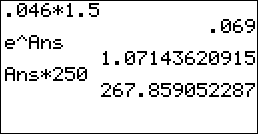<< Previous  Top  |  1 | 2 | 3 | 4 | 5  |  Return to Index

 Cite this article as: Stapel, Elizabeth. "The 'Natural' Exponential 'e'." Purplemath. Available from     https://www.purplemath.com/modules/expofcns5.htm. Accessed [Date] [Month] 2016

Study Skills Survey

Tutoring from Purplemath
Find a local math tutor

 Copyright © 2021  Elizabeth Stapel   |   About   |   Terms of Use   |   Linking   |   Site Licensing Contact Us